Diet problems

Chapter 12 Class 12 Linear Programming
Concept wise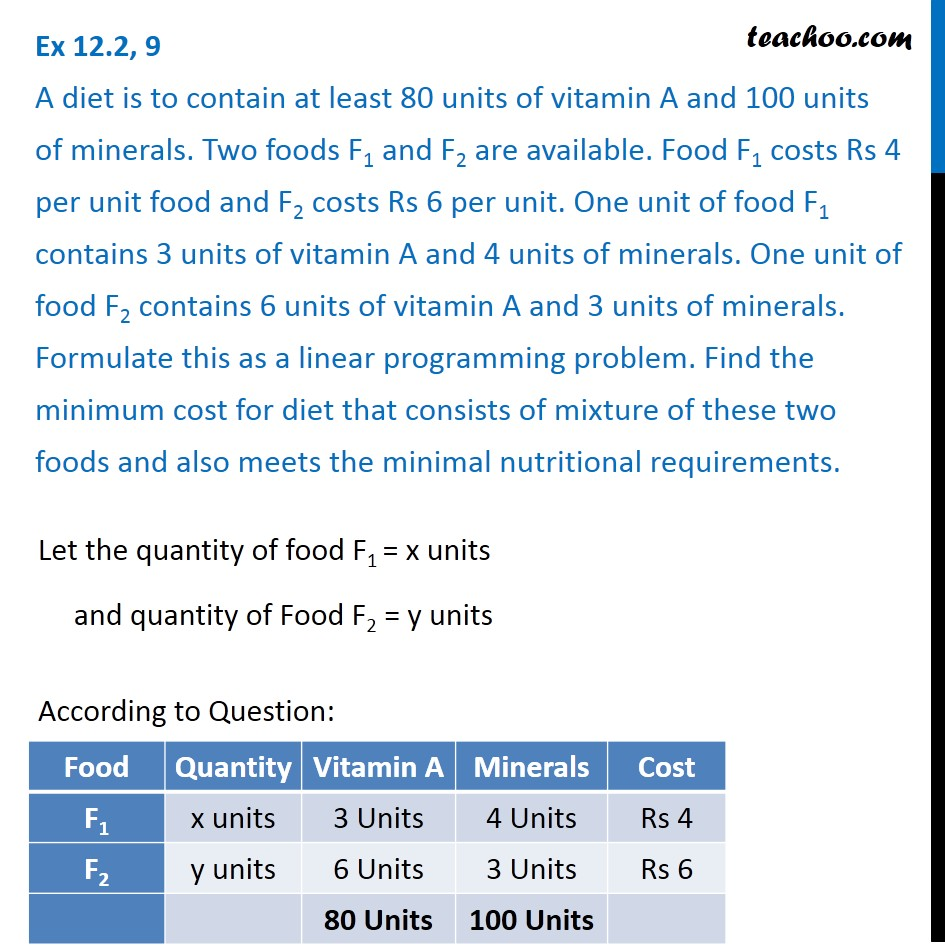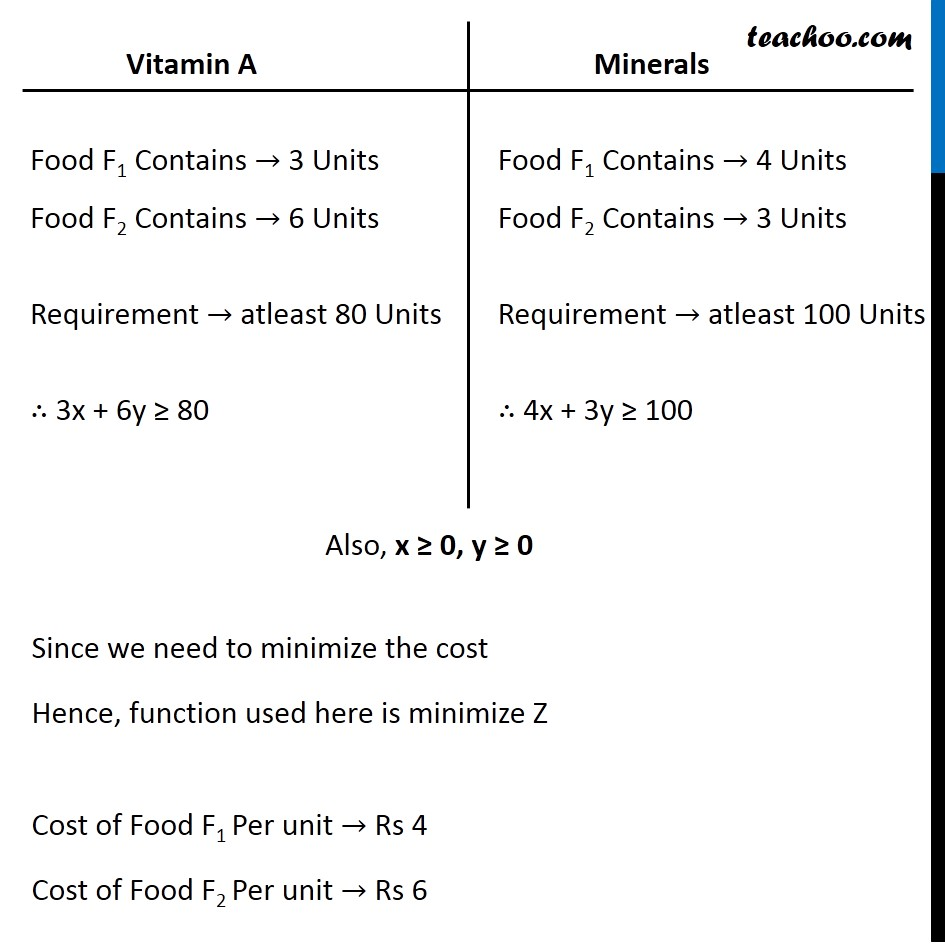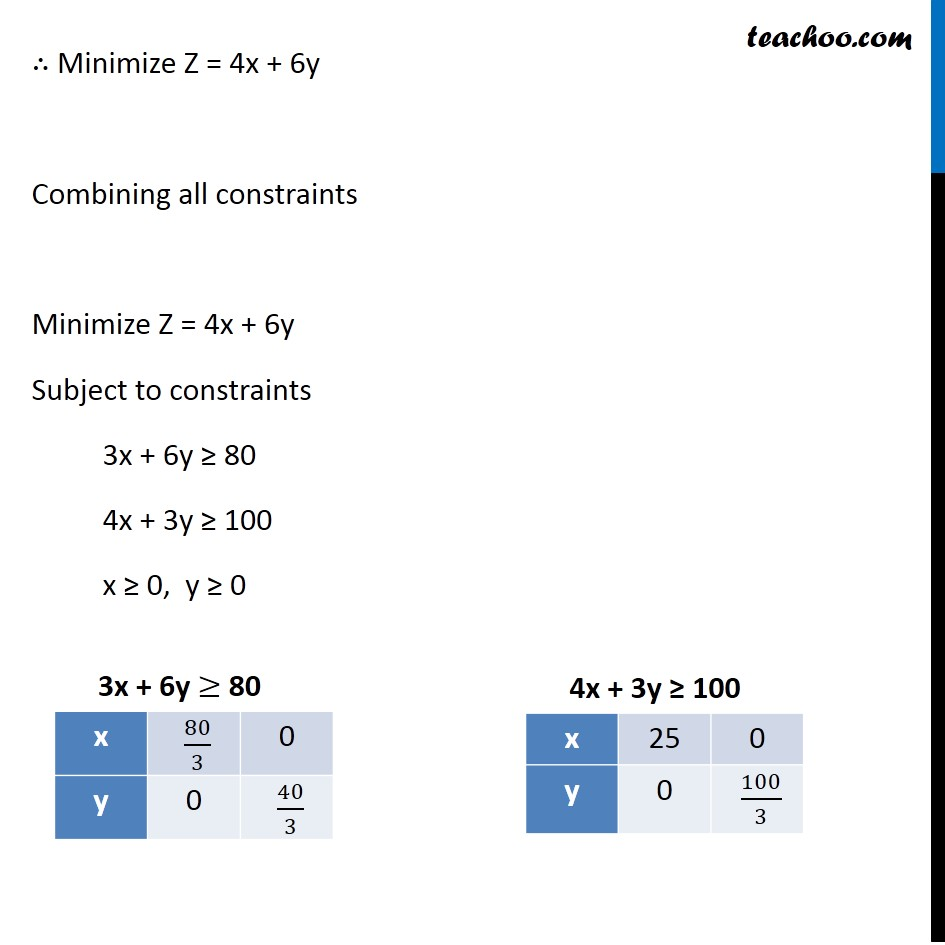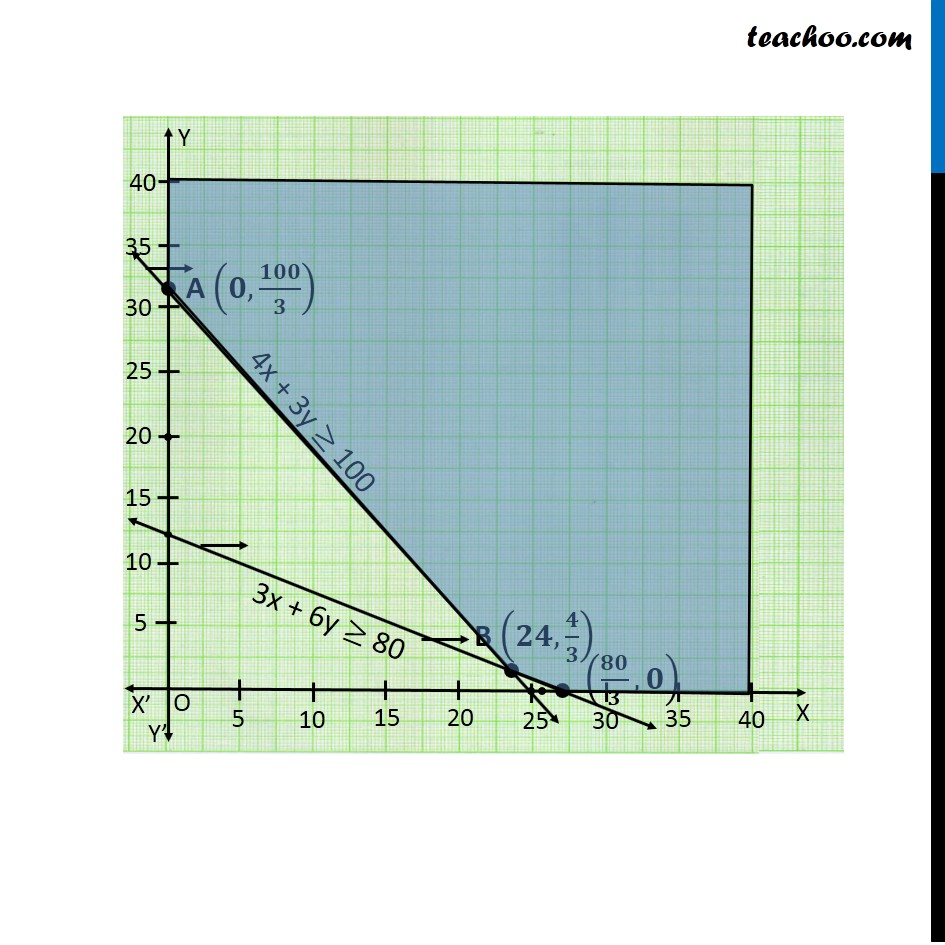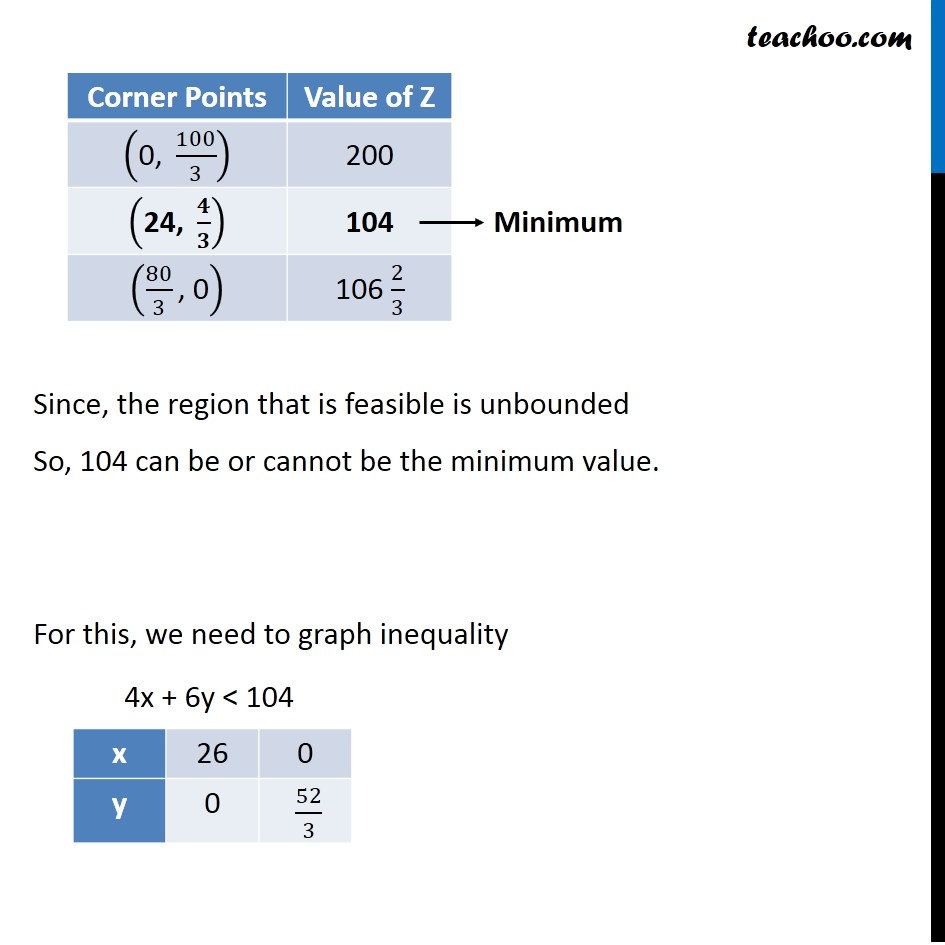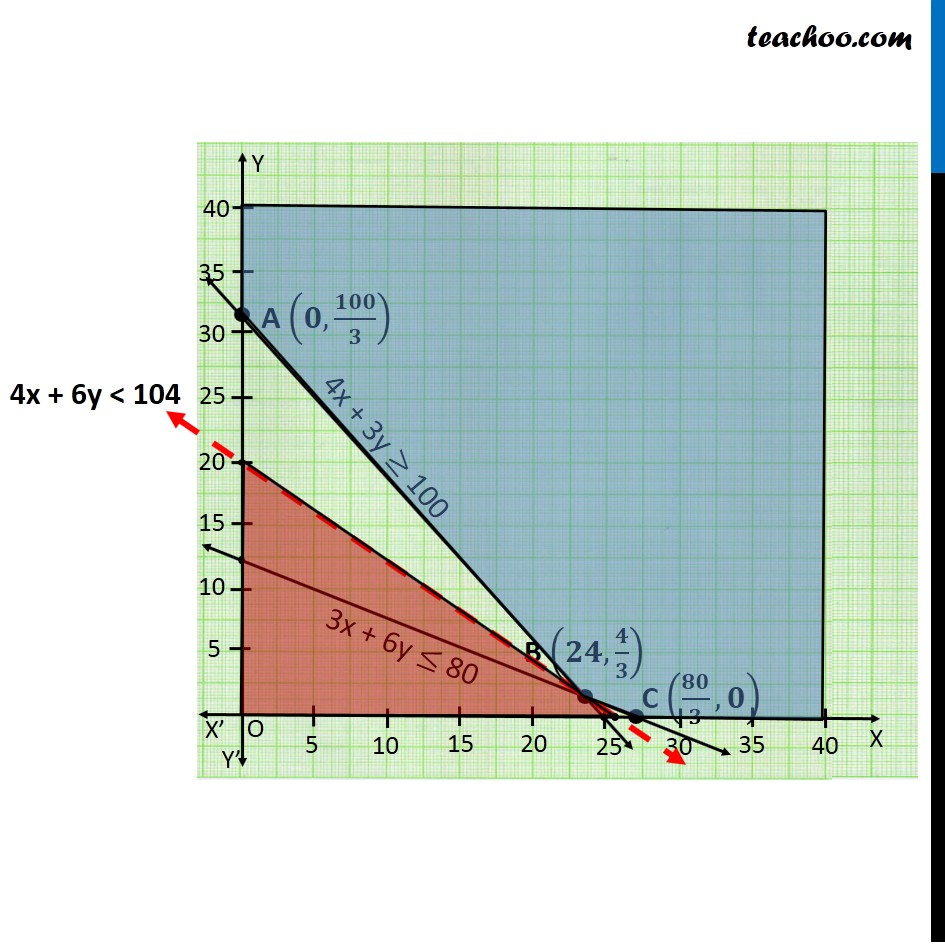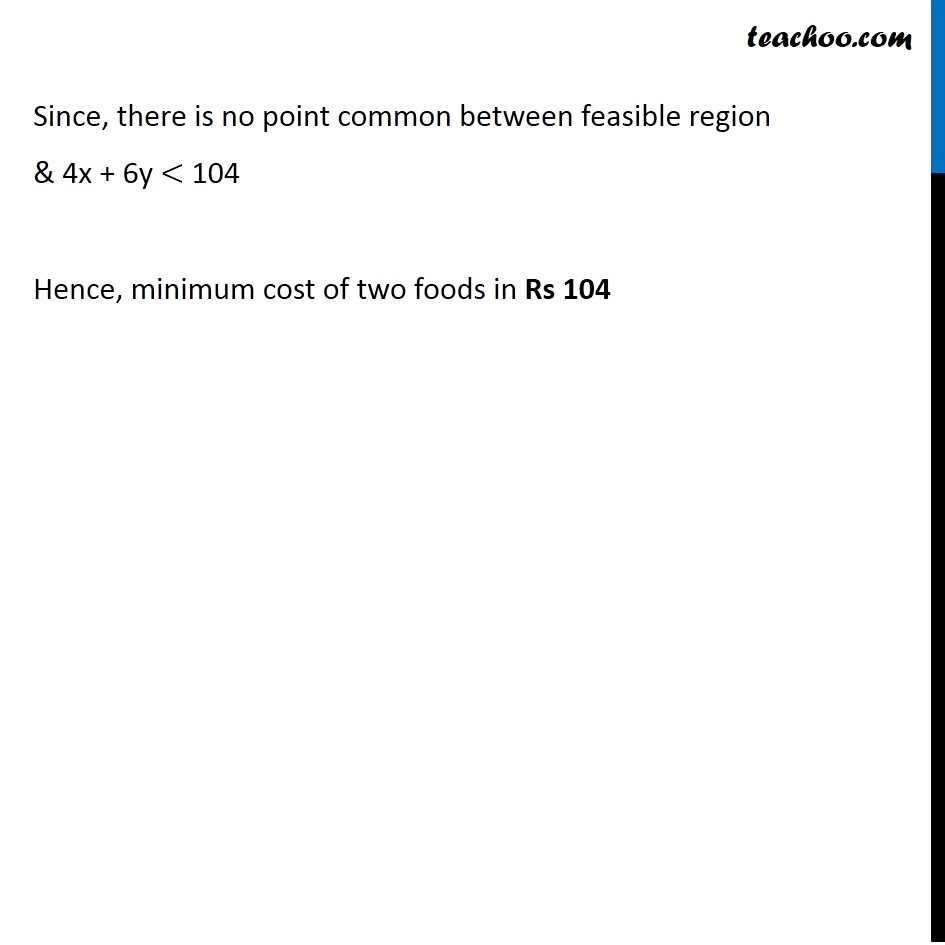Learn in your speed, with individual attention - Teachoo Maths 1-on-1 Class

### Transcript

=Question 9 A diet is to contain at least 80 units of vitamin A and 100 units of minerals. Two foods F1 and F2 are available. Food F1 costs Rs 4 per unit food and F2 costs Rs 6 per unit. One unit of food F1 contains 3 units of vitamin A and 4 units of minerals. One unit of food F2 contains 6 units of vitamin A and 3 units of minerals. Formulate this as a linear programming problem. Find the minimum cost for diet that consists of mixture of these two foods and also meets the minimal nutritional requirements. Let the quantity of food F1 = x units and quantity of Food F2 = y units According to Question: Vitamin A Food F1 Contains → 3 Units Food F2 Contains → 6 Units Requirement → atleast 80 Units ∴ 3x + 6y ≥ 80 Minerals Food F1 Contains → 4 Units Food F2 Contains → 3 Units Requirement → atleast 100 Units ∴ 4x + 3y ≥ 100 Since we need to minimize the cost Hence, function used here is minimize Z Cost of Food F1 Per unit → Rs 4 Cost of Food F2 Per unit → Rs 6 ∴ Minimize Z = 4x + 6y Combining all constraints Minimize Z = 4x + 6y Subject to constraints 3x + 6y ≥ 80 4x + 3y ≥ 100 x ≥ 0, y ≥ 0 Since, the region that is feasible is unbounded So, 104 can be or cannot be the minimum value. For this, we need to graph inequality Since, there is no point common between feasible region & 4x + 6y < 104 Hence, minimum cost of two foods in Rs 104